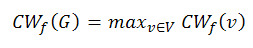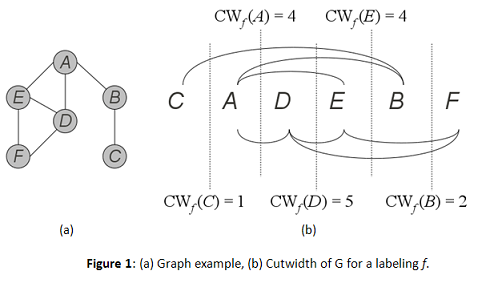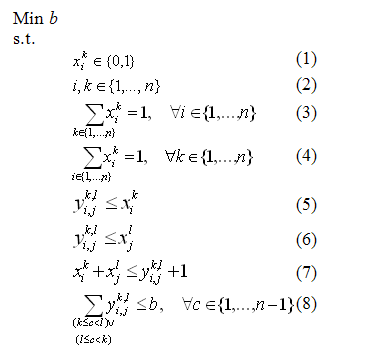# Cutwidth Minimization Problem

Martí R., Pantrigo J.J., Duarte A. and Pardo E.G. (2010)
Optsicom project, University of Valencia (Spain)

# Problem Description

The Cutwidth Minimization Problem (CMP) is an NP-hard problem (Gavril 1977) and consists of finding a linear layout of a graph so that the maximum linear cut of edges (i.e., the number of edges that cut a line between consecutive vertices) is minimized. The cutwidth minimization problem can be easily described in mathematical terms. Given a graph G=(V,E) with n=|V| and m=|E|, a labeling or linear arrangement f of G assigns the integers {1,2,…,n} to the vertices in V, where each vertex receives a different label. The cutwidth of a vertex v with respect to f, CWf(v), is the number of edges (u,w) ∈ E satisfying f(u)≤f(v)<f(w). The cutwidth of the graph, CWf(G), is the maximum of the cutwidth of its vertices:Figure 1.a shows an example of an undirected graph with 6 vertices and 7 edges. Figure 1.b shows a labeling, f, of the graph in Figure 1.a, setting the vertices in a line with the order of the labeling, as commonly represented in the CMP. In this way, since f(C)=1, vertex C comes first, followed by vertex A (f(A)=2) and so on. We represent f with the ordering (C, A, D, E, B, F) meaning that vertex C is located in the first position (label 1), vertex A is located in the second position (label 2) and so on. In Figure 1.b, the cutwidth of each vertex is represented as a dashed line with its corresponding value. For example, the cutwidth of vertex C is CWf(C) = 1, because the edge (C,B) has and endpoint in C labeled with 1 and the other endpoint in a vertex labeled with a value larger than 1. In a similar way, we can compute the cutwidth of vertex A, CWf(A)=4, by counting the appropriate number of edges ((C,B), (A,B), (A,E), and (A,D)). Then, since the cutwidth of the graph G, CWf(G), is the maximum of the cutwidth of all vertices in V, in this particular example we obtain CWf(G)=CWf(D)=5.The CMP can be formulated as 0/1 linear integer programming problem as follows (Luttamaguzi et al., 2005):where xik is a decision binary variable whose indices are i, k ∈ {1,2,...,n}. This variable specifies whether i is placed in position k in the ordering. In other words, for all xik (i, k={1, 2,...,n})they take on value 1 if and only if i occupies the position k in the ordering; otherwise xik takes on value 0. Constraints (3) and (4) ensure that each vertex is only assigned to one position and one position is only assigned to one vertex respectively. Consequently, contraints (1), (2), (3) and (4) together implies that a solution of the problem is an ordering.

The decision binary variable yikjl is defined as xikxjl, where i, j ∈ {1,2,...,n}, (vi, vj) ∈ E and k, l ∈ {1,2,...,n} the labels associated to vertex vi and vj respectively. In the linear formulation above this conjunction is computed with constraints (5), (6) and (7).

Constraint (8) computes for each position c in the ordering, the number of edges whose origin is placed in any position k (1 ≤ k < c) and destination in any position l (c < ln). The cutwidth problem consists of minimizing the maximum number of cutting edges in any position, c ∈{1,...,n - 1} of the labeling. Therefore, the objective function b must be larger than or equal to this quantity.

# State of the Art Methods

The most relevant heuristic methods developed to solve this problem are:

• GRASP+Evolutionary Path Relinking: Hybrid method that combines GRASP methodology with Evolutionary Path-Relinking. Andrade and Resende (2007b).
• Simulated Annealing : Simulated Annealing based on different constructive methods and local search procedures. Cohoon and Sahni (1987)
• Scatter Search : Scatter Search method based on a GRASP constructive algorithm, a local search strategy based on insertion moves and voting-based combination methods. Pantrigo et al. (2012).
• Tabu Search : A Tabu Search method used to help a Branch-and-bound algorithm. Palubeckis and Rubliauskas (2012).
• Variable Neighbourhood Search : A new variant of the Variable Neighbourhood Search framework called Variable Formulation Search for the Cutwidth Minimization Problem. Pardo et al. (2013). (Results)

# Instances (CMPLIB)

We have compiled three sets of instances for our experimentation, totalizing 252 instances. The first one, Small, was introduced in Martí et al. (2008), the second one, Grids, was introduced in Rolim et al. (1995) and the third one, Harwell-Boeing, is a subset of the public-domain Matrix Market library (available at http://math.nist.gov/MatrixMarket/data/Harwell-Boeing/). Next, it can be found a brief description of the origin and characteristics of the sets of instances:

• Small: This data set consists of 84 graphs introduced in the context of the bandwidth reduction problem. The number of vertices ranges from 16 to 24, and the number of edges ranges from 18 to 49.
• Grids: This data set consists of 81 matrices constructed as the Cartesian product of two paths (Raspaud et al., 2009). They are also called two dimensional meshes and, as documented in Raspaud et al. (2009), the optimal solution of the cutwidth problem for these types of instances is known by construction. For this set of instances, the vertices are arranged on a grid with a dimension width x height where width, height are selected from the set {3, 6, 9, 12, 15, 18, 21, 24, 27}.
• Harwell-Boeing: We derived 87 instances from the Harwell-Boeing Sparse Matrix Collection. This collection consists of a set of standard test matrices M = (Mij) arising from problems in linear systems, least squares, and eigenvalue calculations from a wide variety of scientific and engineering disciplines. Graphs are derived from these matrices by considering an edge (i,j) for every element Mij ≠ 0. From the original set we have selected the 87 graphs with n ≤ 700. Their number of vertices ranges from 30 to 700 and the number of edges from 46 to 41686.

The CMPLIB contains 252 instances:

# Computational Experience

We have performed an intensive experimentation with the best know methods to compute the best known values for the instances. In addition, we have performed a computational comparison of the state of the art methods on the reported instances. The experiment was performed in a Intel Core 2 Quad CPU Q 8300 with 6 GiB of RAM and Ubuntu 9.04 64 bits OS. Results and best known values for instances can be reviewed in Pantrigo et al. (2010) and Martí et al. (2010) and can be downloaded here.

# References

• Andrade, D.V. and M.G.C. Resende. GRASP with path-relinking for network migration scheduling. Proceedings of International Network Optimization Conference, 2007a.
• Andrade, D.V. and M.G.C. Resende. GRASP with evolutionary path-relinking.Proceedings of Seventh Metaheuristics International Conference (MIC), 2007b.
• Cohoon, J. and S. Sahni. Heuristics for the Board Permutation Problem.Journal of VLSI and Computer Systems, 2, 37- 61, 1987.
• Gavril, F. Some NP-complete problems on graphs. In Proceedings of the 11th conference on information Sciences and Systems, 91-95, 1977.
• Luttamaguzi, J., M. Pelsmajer, Z. Shen and B. Yang. Integer Programming Solutions for Several Optimization Problems in Graph Theory. Technical report, DIMACS, 2005.
• Martí, R., V. Campos and E. Piñana, Branch and Bound for the Matrix Bandwidth Minimization. European Journal of Operational Research, 186:513-528, 2008.
• Martí, R., J.J. Pantrigo, A. Duarte and E.G. Pardo. Branch and Bound for Cutwidth Minimization Problem. Computers & Operations Research. Volume 40, Issue 1, Pages 137–149, 2013
• Palubeckis, G., D. Rubliauskas. A branch-and-bound algorithm for the minimum cut linear arrangement problem. Journal of Combinatorial Optimization. Volume 24, Issue 4, Pages 540-563, 2012
• Pantrigo, J.J., R. Martí, A. Duarte and E.G. Pardo. Scatter Search for the Cutwidth Minimization Problem Annals of Operations Research. Volume 199, Issue 1, Pages 285-304, 2012.
• Pardo, E.G., Mladenovic N., Pantrigo, J.J., Duarte A. Variable Formulation Search for the Cutwidth Minimization Problem. Applied Soft Computing. Volume 13, Issue 5, Pages 2242–2252, 2013.
• Raspaud, A., H. Schröder, O. Sýkora, L. Török, and I. Vrt'o. Antibandwidth and cyclic antibandwidth of meshes and hypercubes. Discrete Mathematics. 309:3541-3552, 2009.
• Rolim, J., O. Sýkora and I. Vrt'o. Cutwidth of the de Bruijn graph. RAIRO Informatique Théorique et Applications, 29(6):509-514, 1995.